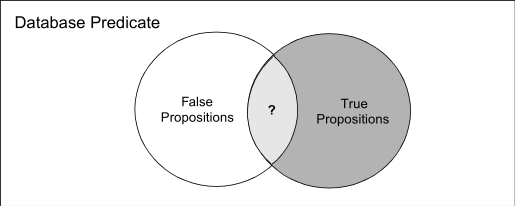# Bivalent and Higher-Valued Logics | Database Design | Teradata Vantage - 17.10 - Bivalent and Higher-Valued Logics - Advanced SQL Engine - Teradata Database

## Teradata Vantage™ - Database Design

Product
Release Number
17.10
Published
July 2021
Language
English (United States)
Last Update
2021-07-27
dita:mapPath
kko1591750222108.ditamap
dita:ditavalPath
kko1591750222108.ditaval
dita:id
zqc1472244571611

This topic presents a brief overview of the relevant predicate logic underlying the database relational model and the place of nulls in that logic.

A two-valued logic (2VL) has 2 truth values: TRUE and FALSE. while a multivalued logic (MVL) has the truth values TRUE and FALSE plus an additional number, where the number of truth values depends on the individual logic.

## First Order Predicate Logic and Bivalent Logic

The classic first order predicate logic is based on bivalent logic (a bivalent, or Boolean, logic has exactly two truth values: TRUE and FALSE, as noted in the previous topic. Higher-valued logics have three or more truth values. For example, SQL uses a 3VL having the following three truth values: TRUE, FALSE, and UNKNOWN).

Upon close examination, it is apparent that the logic supporting SQL is actually a bivalent logic (2VL) with additional ad hoc support for nulls. Note that the inconsistent treatment of missing values in SQL is common to all vendors: it is not an implementation problem per se, but rather a problem with the way SQL itself is defined.

## The Closed World Assumption Revisited

Recall that any tuple in a relation body is assumed to conform to the Closed World Assumption (see The Closed World Assumption). With the addition of nulls to the database relational model, the CWA can no longer be assumed to be true. As an example, consider the following Venn diagram.In contrast with the Venn diagram in the topic The Closed World Assumption, there is overlap between the set of false propositions and the set of true propositions, represented by the darker shading. Better put, there is potential overlap between those sets. For example, suppose the employee table has an emp_age column that permits nulls. The company hires an employee who does not disclose her age in the application form. All other information for the employee is entered.

Because the age for this employee is missing, the tuple that represents her can neither be evaluated as true nor as false, so it falls into the ambiguous intersection of the Venn diagram.

At some point, it is discovered that this employee is 15 years old, which violates a constraint on the emp_age column that requires all employees to be at least 18 years old. The proposition for this employee now evaluates as FALSE, and the tuple that represents her is no longer valid in the database. Nevertheless, that tuple was treated as valid from the time its data was entered until the time the age of the employee was discovered to violate the constraint specified for the emp_age column, and that information was used to produce any number of reports which are, in retrospect, known to be non-valid.

## Number of Logical Operators Supported for Bivalent and Trivalent Logics

In a bivalent logic, there are exactly four possible monadic, or single-operand, logical operators, as indicated in the following table. Note that the numberings have no meaning other than to distinguish the operators from one another.

This operator number … Performs these mappings of TRUE and FALSE values …
1 T →  T

F  T

2 T  F

F  F

3 T  F

F  T

4 T →  T

F  F

By generalization, it is also true that there are exactly 16 possible dyadic, or two-operand, logical operators.

The formulas that produce the numbers of monadic and dyadic operators are provided as follows, where n represents the valence of the logic in question. For a bivalent logic, its value is 2; for a trivalent logic, its value is 3.

Number of monadic operators = n n

Number of dyadic operators = n n 2

By performing the calculations for a trivalent logic, you find that 3VLs have 27 possible monadic operators and 19,683 possible dyadic operators. These figures, of course, are redundant: they represent the number of possible operators, not the number of useful operators.

SQL, like most programming languages, overdetermines its operators. For example, the function performed by the = operator in the following two equations is not identical internally, but the language uses the same symbol for the operation in both cases.

• ‘value’ = ‘value’
• 2 = 2

The issue raised here is whether SQL supports a sufficient number of trivalent logical operators to fully support a 3VL, and the answer to that question is negative.

Nevertheless, they indicate that SQL does not support anywhere near the number of logical operators required to support a coherent 3VL.

## SQL Support for a Consistent Trivalent Logic

The treatment of nulls by SQL is not only inconsistent, but it is also sometimes incorrect from a real world perspective.

The purpose of this description of the problems inherent in SQL missing values is to help you to understand what the most important inconsistencies are and how you can avoid some of them through careful database design.